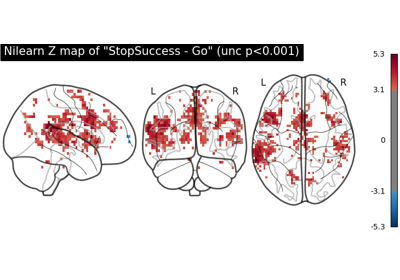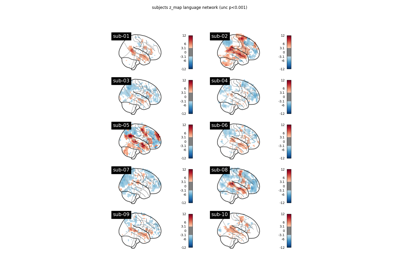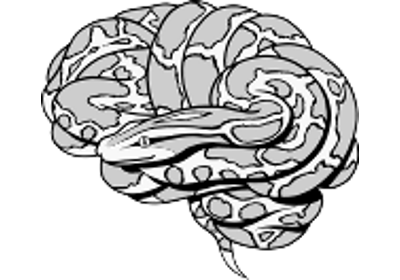Note

This page is a reference documentation. It only explains the function signature, and not how to use it. Please refer to the user guide for the big picture.

# 8.12.15.4. nilearn.glm.first_level.first_level_from_bids¶

nilearn.glm.first_level.first_level_from_bids(dataset_path, task_label, space_label=None, img_filters=None, t_r=None, slice_time_ref=0.0, hrf_model='glover', drift_model='cosine', high_pass=0.01, drift_order=1, fir_delays=, min_onset=- 24, mask_img=None, target_affine=None, target_shape=None, smoothing_fwhm=None, memory=Memory(location=None), memory_level=1, standardize=False, signal_scaling=0, noise_model='ar1', verbose=0, n_jobs=1, minimize_memory=True, derivatives_folder='derivatives')[source]

Create FirstLevelModel objects and fit arguments from a BIDS dataset.

It t_r is not specified this function will attempt to load it from a bold.json file alongside slice_time_ref. Otherwise t_r and slice_time_ref are taken as given.

Parameters
dataset_pathstr

Directory of the highest level folder of the BIDS dataset. Should contain subject folders and a derivatives folder.

space_labelstr, optional

Specifies the space label of the preprocessed bold.nii images. As they are specified in the file names like _space-<space_label>_.

img_filterslist of tuples (str, str), optional

Filters are of the form (field, label). Only one filter per field allowed. A file that does not match a filter will be discarded. Possible filters are ‘acq’, ‘ce’, ‘dir’, ‘rec’, ‘run’, ‘echo’, ‘res’, ‘den’, and ‘desc’. Filter examples would be (‘desc’, ‘preproc’), (‘dir’, ‘pa’) and (‘run’, ‘10’).

derivatives_folderstr, optional

derivatives and app folder path containing preprocessed files. Like “derivatives/FMRIPREP”. Default=”derivatives”.

All other parameters correspond to a `FirstLevelModel` object, which
contains their documentation. The subject label of the model will be
determined directly from the BIDS dataset.
Returns
modelslist of FirstLevelModel objects

Each FirstLevelModel object corresponds to a subject. All runs from different sessions are considered together for the same subject to run a fixed effects analysis on them.

models_run_imgslist of list of Niimg-like objects,

Items for the FirstLevelModel fit function of their respective model.

models_eventslist of list of pandas DataFrames,

Items for the FirstLevelModel fit function of their respective model.

models_confoundslist of list of pandas DataFrames or None,

Items for the FirstLevelModel fit function of their respective model.

## 8.12.15.4.1. Examples using `nilearn.glm.first_level.first_level_from_bids`¶